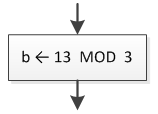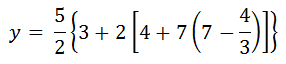Every high-level programming language supports arithmetic operators as shown in the following table.

## PHP

 Arithmetic Operator Description + Addition – Subtraction * Multiplication / Division % Modulus (remainder after integer division)

The first four operators are straightforward and need no further explanation.

## Java, C++, C#

 Arithmetic Operator Description + Addition – Subtraction * Multiplication / Division % Modulus (remainder after integer division)

The first four operators are straightforward and need no further explanation.

## Visual Basic

 Arithmetic Operator Description + Addition – Subtraction * Multiplication / Division \ Quotient after integer division `Mod` Remainder after integer division (Modulus) ^ Exponentiation

The first four operators are straightforward and need no further explanation.

The integer division operator returns the quotient of an integer division, which means that the statement

`a = 13 \ 3`

assigns a value of 4 to variable a.

## Python

 Arithmetic Operator Description + Addition – Subtraction * Multiplication / Division // Quotient after integer division % Remainder after integer division (Modulus) ** Exponentiation

The first four operators are straightforward and need no further explanation.

The integer division operator returns the quotient of an integer division, which means that the statement

`a = 13 // 3`

assigns a value of 4 to variable a.

The modulus operator returns the remainder of an integer division, which means that the statement

## PHP

`\$a = 13 % 3;`

## Java, C++, C#

`a = 13 % 3;`

## Visual Basic

`a = 13 Mod 3`

## Python

`a = 13 % 3`

assigns a value of 1 to variable` a`.

Notice: Please keep in mind that flowcharts are a loose method used to represent an algorithm. Although the use of the modulus ( % ) operator is allowed in flowcharts, this book uses the commonly accepted MOD operator instead! For example, the PHP statement` \$b = 13 % 3` is represented in a flowchart asIn mathematics, as you already know, you are allowed to use parentheses as well as braces and brackets.However, in most programming languages there is no such thing as braces and brackets. Parentheses are all you have; therefore, the same expression should be written using parentheses instead of braces or brackets.

## PHP

`\$y = 5 / 2 * (3 + 2 * (4 + 7 * (7 – 4 / 3)));`

## Java, C++, C#

`y = 5 / 2 * (3 + 2 * (4 + 7 * (7 – 4 / 3)));`

## Visual Basic

`y = 5 / 2 * (3 + 2 * (4 + 7 * (7 – 4 / 3)))`

## Python

`y = 5 / 2 * (3 + 2 * (4 + 7 * (7 – 4 / 3)))`

One other thing that is legal in mathematics but not in most programming languages is that you can skip the multiplication operator and write 3x, meaning “3 times x. In a programming language, however, you must always use an asterisk anywhere a multiplication operation exists. This is one of the most common mistakes novice programmers make when they write mathematical expressions in a programming language.